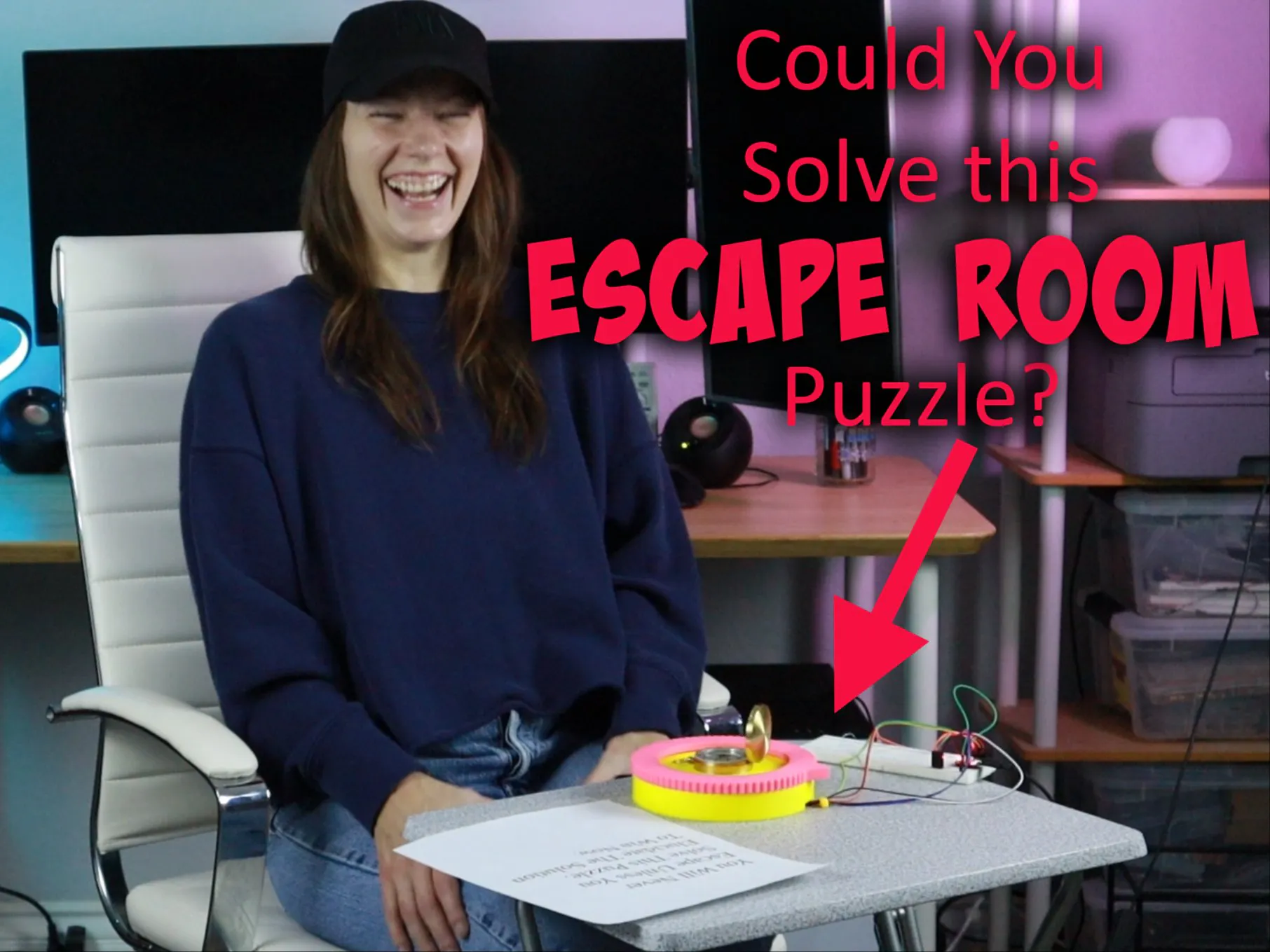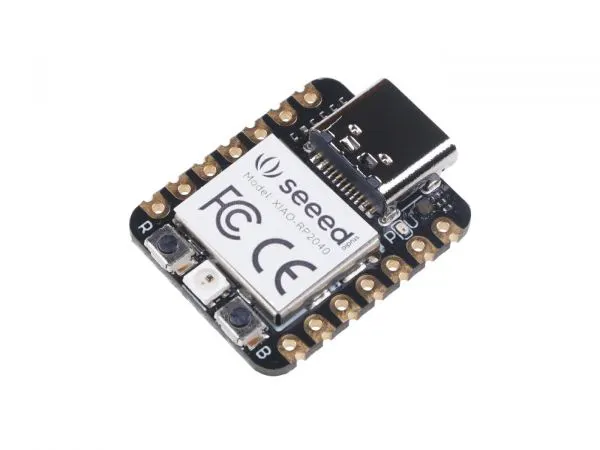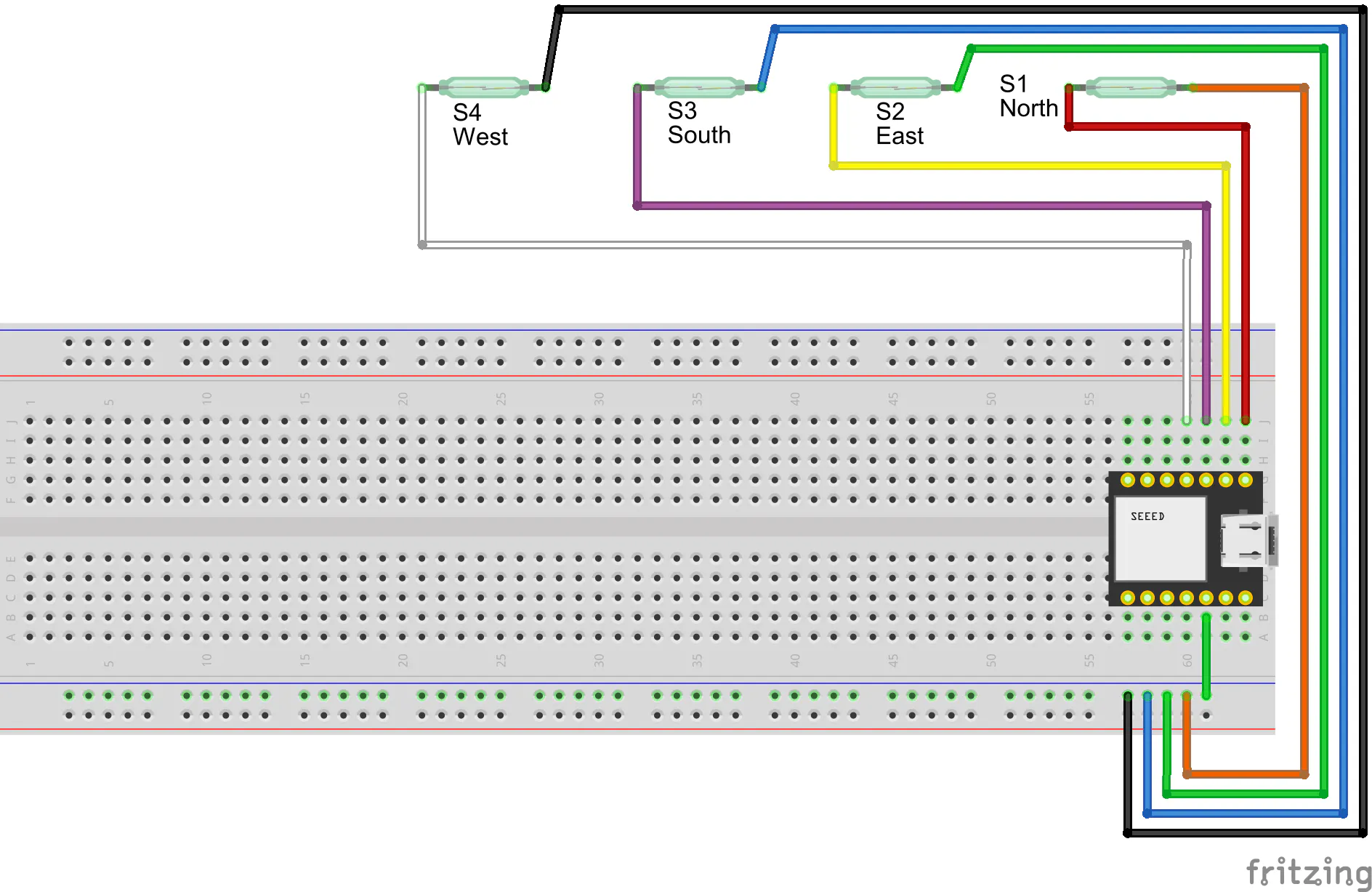Created November 13, 2023 © CC BY-NC-SA

# Compass Lock Escape Room Puzzle

A puzzle for escape rooms that players solve by turning a dial to cardinal directions according to a secret sequence.

BeginnerFull instructions provided1 hour1## Things used in this project

### Hardware componentsSeeed Studio Seeed XIAO RP2040
×1Reed Switch, SPST-NO
×4

### Software apps and online servicesArduino IDE

### Hand tools and fabrication machines3D Printer (generic)

## Schematics

### Wiring schematic## Code

### Arduino Code

Arduino
```#include <Adafruit_NeoPixel.h>

#define LED_PIN 12                                    // the pin for the onboard RGB LED
#define LED_POWER 11                                  // the pin that controls power to the onboard LED
#define NUMPIXELS 1                                   // we only have one "pixel" (LED)
#define N_PIN A0                                      // Reed switch on the North position
#define E_PIN A1                                      // Reed switch on the East position
#define S_PIN A2                                      // Reed switch on the South position
#define W_PIN A3                                      // Reed switch on the West position

Adafruit_NeoPixel pixels(NUMPIXELS, LED_PIN, NEO_GRB + NEO_KHZ800);

int lastDir = 5;                                      // Save the last registered position (N=0, E=1, S=2, W=3)
int curDir = 5;                                       // Save the current registered position

const int code[] = {3, 0, 1, 2, 1, 2, 3, 0};          // the solution sequence (you can change this)
int inSequence[] = {5, 5, 5, 5, 5, 5, 5, 5};          // stores the sequence entered by the player
const int codeLength = 8;                             // the sequence length (needs to match both array above)

void setup() {
Serial.begin(115200);

pixels.begin();
pinMode(LED_POWER,OUTPUT);
digitalWrite(LED_POWER, HIGH);                      // turn on power to the onboard LED
pixels.clear();
pixels.setPixelColor(0, pixels.Color(127, 0, 0));   // set onboard LED to red
pixels.show();

pinMode(E_PIN, INPUT);
pinMode(S_PIN, INPUT);
pinMode(W_PIN, INPUT);

}

void loop() {
if (analogRead(N_PIN) > 1015){                      // check each pin to see if it exceeds the threshold, indicating that the swith is "on"
curDir = 0;                                       // save the current position
} else if (analogRead(E_PIN) > 1015){
curDir = 1;
} else if (analogRead(S_PIN) > 1015){
curDir = 2;
} else if (analogRead(W_PIN) > 1015){
curDir = 3;
}

if (lastDir != curDir){                             // check if current position has changed
lastDir = curDir;
Serial.println(lastDir);
pushToEnd(inSequence, lastDir, codeLength);       // add the new position to the sequence
}

winCheck();                                         // check if they've solved the puzzle

delay(100);

}

void pushToEnd(int a[], int newVal, int length) {
// this function takes the sequence array, the value for the new position,
// and the length of the sequence array. It then pushes all of the current
// sequence values forward by one position, then adds the new position
// value to the end.

int b[length];

for (int i = 0; i < (length - 1); i++){
b[i] = a[i + 1];
}
b[length - 1] = newVal;

for (int i = 0; i < (length); i++){
a[i] = b[i];
}

Serial.print(a);
Serial.print(", ");
Serial.print(a);
Serial.print(", ");
Serial.print(a);
Serial.print(", ");
Serial.print(a);
Serial.print(", ");
Serial.print(a);
Serial.print(", ");
Serial.print(a);
Serial.print(", ");
Serial.print(a);
Serial.print(", ");
Serial.println(a);
}

void winCheck() {
// this function checks to see if the player solved the puzzle,
// by looking at both arrays and checking if the values in each
// index position match. Each match adds 1 to the "score". If
// that score equals the length of the sequence array, we know
// all numbers match and they've won.

int score = 0;
for (int i = 0; i < codeLength; i++){
if (code[i] == inSequence[i]){
score++;
}
}

if (score >= codeLength){

for (int i = 0; i < codeLength; i++){
inSequence[i] = 5;
}

Serial.println("Solved!");

// What to do when they win:
pixels.clear();
pixels.setPixelColor(0, pixels.Color(0, 127, 0));
pixels.show();
// you can replace that with something like a function
// call for opening a lock.

}
}
```

## Credits

### Cameron Coward

14 projects • 1257 followers
Writer for Hackster News. Proud husband and dog dad. Maker and serial hobbyist.